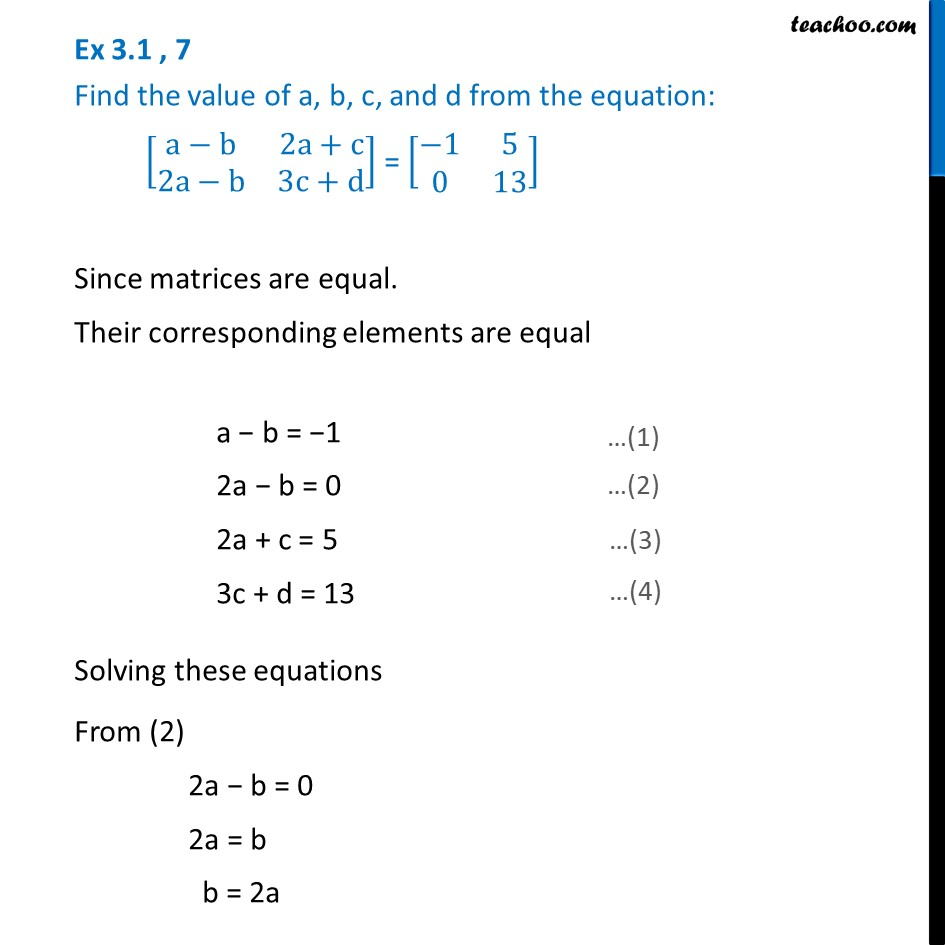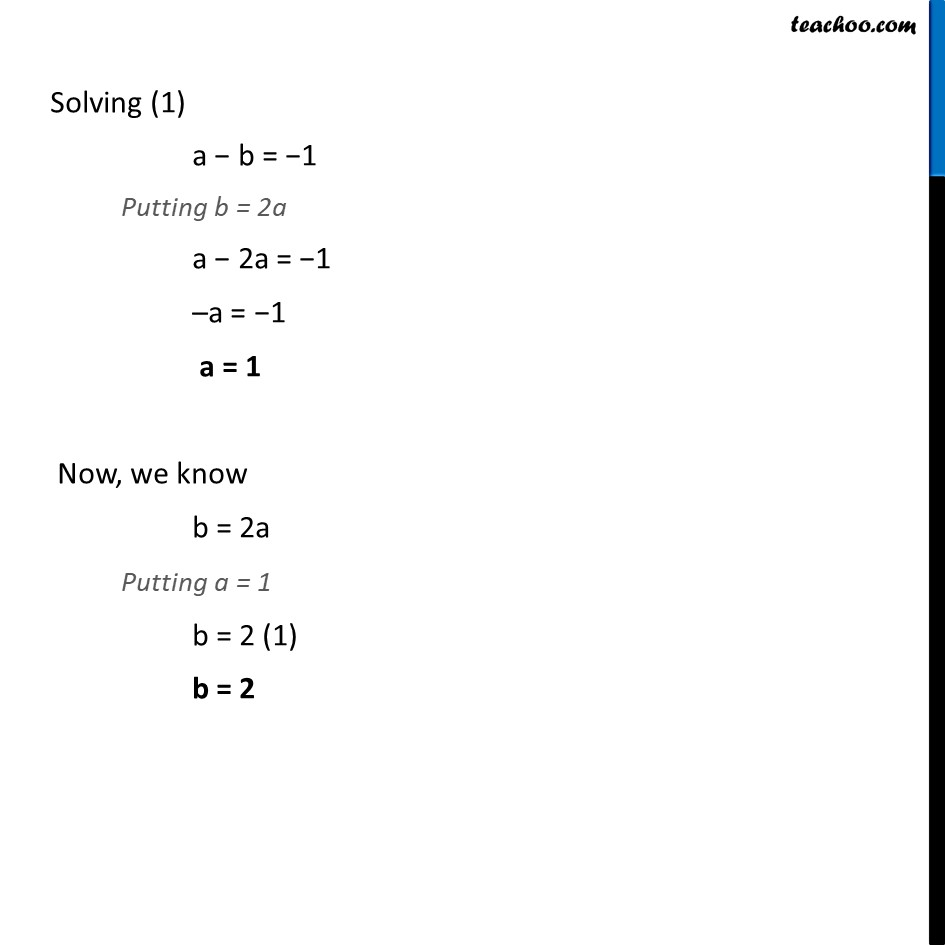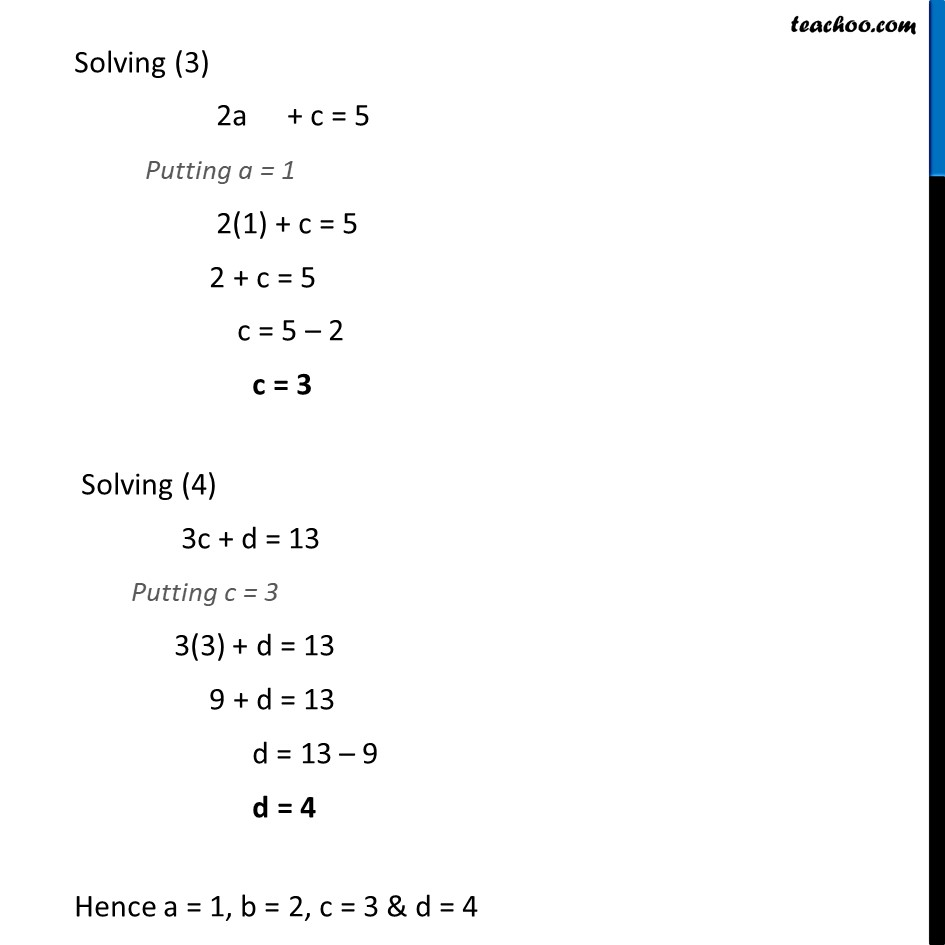Ex 3.1

Chapter 3 Class 12 Matrices
Serial order wiseLearn in your speed, with individual attention - Teachoo Maths 1-on-1 Class

### Transcript

Ex 3.1 , 7 Find the value of a, b, c, and d from the equation: [■8(a−b&2a+c@2a−b&3c+d)] = [■8(−1&5@0&13)] Since matrices are equal. Their corresponding elements are equal a − b = −1 2a − b = 0 2a + c = 5 3c + d = 13 Solving these equations From (2) 2a − b = 0 2a = b b = 2a Solving (1) a − b = −1 Putting b = 2a a − 2a = −1 –a = −1 a = 1 Now, we know b = 2a Putting a = 1 b = 2 (1) b = 2 Solving (3) 2a + c = 5 Putting a = 1 2(1) + c = 5 2 + c = 5 c = 5 – 2 c = 3 Solving (4) 3c + d = 13 Putting c = 3 3(3) + d = 13 9 + d = 13 d = 13 – 9 d = 4 Hence a = 1, b = 2, c = 3 & d = 4